# Curse of dimension

curse of dimensionality

Many high-dimensional problems are difficult to solve for any numerical method (algorithm). Their complexity, i.e., the cost of an optimal algorithm, increases exponentially with the dimension. This is proved by providing exponentially large lower bounds that hold for all algorithms. Many problems suffer from the curse of dimension. Examples include numerical integration, optimal recovery (approximation) of functions, global optimization, and solution of integral equations and partial differential equations. It does not, however, hold for convex optimization and ordinary differential equations. The term "curse of dimensionality" seems to go back to R. Bellman [a2].

The investigation of the curse of dimension is one of the main fields of information-based complexity, see also Optimization of computational algorithms. Whether a problem suffers from the curse of dimension depends on the exact definition of the norms and function classes that are studied. Some high-dimensional problems can be computed efficiently using the Smolyak algorithm.

The curse of dimension typically happens in the worst-case setting, where the error and cost of algorithms are defined by their worst performance. One can hope to break the curse of dimension by weakening the worst-case assurance and switching to the randomized setting (see also Monte-Carlo method) or to the average-case setting (see Bayesian numerical analysis).

Below, results for numerical integration are discussed in more detail. Many of the results are similar for other problems such as approximation (optimal recovery) of functions, solution of partial differential equations and integral equations.

## Worst-case setting.

Consider a quadrature formula of the form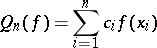(a1)

with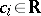and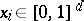for the approximation of the integralLet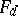be a class of integrable functions on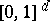. Below,will be the unit ball in a suitable Banach space. The (worst case) error of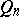onis defined byand one also definesThe number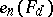is the (worst case) error of the optimal cubature formula (for the class) when at mostsample points are used. It is known that formulas of the form (a1) are general enough. Indeed, non-linear methods and adaptive quadrature do not yield better estimates ifis convex and symmetric. Hence, one may say that the-complexity of the problem is given by the numberMuch is known concerning the order ofand of related numbers (such as widths and-entropy (see also Width;-entropy; or [a9]), for many different). Classical results on the order, see (a2) and (a3) below, contain unknown constants that depend onand therefore cannot always answer the question whether there is a curse of dimension or not.

Two specific results on the order ofare as follows. Let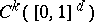be the classical space with norm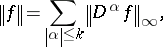where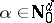and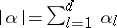, and let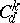be the unit ball of. It is known [a1] that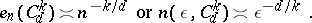(a2)

Hence, integration for the classsuffers from the curse of dimension. Define now a tensor product norm byfor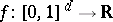, where the sum is over allwith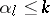for all. This is the space of functions with bounded mixed derivatives. Let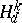be the respective unit ball. It is known [a8] that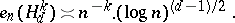(a3)

This result of course implies an upper bound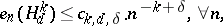(a4)

for all, hence the order of convergence does not depend on. Nevertheless, this problem suffers from the curse of dimension, see [a13].

The lack of the curse of dimension is related to the notion of tractability for continuous problems which was studied by H. Woźniakowski and others, see [a6], [a7], [a11], [a12]. The problem is tractable (inand) if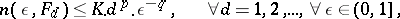for some positive constants,and.

There are general results characterizing which problems are tractable. In particular, this holds for weighted tensor product norms, see [a7], [a13], under natural conditions on weights. The weighted norms correspond to the common fact that for high-dimensional problems usually some variables are "more important" than others. The classical discrepancy also corresponds to a space of functions with bounded mixed derivatives, see [a4]. The respective integration problem is tractable, with upper bounds of the form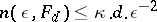, see [a13].

## Randomized setting.

In the randomized setting one considers quadrature formulas (a1) where the sample pointsand the coefficients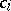are random variables. The error of a randomized method can be defined bywhere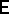stands for mathematical expectation, and one also considers the minimal randomized error, see [a1], [a3], [a5], [a10], [a12], where more general notions are discussed. Instead of (a2), one now has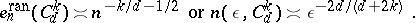The classical Monte-Carlo method gives an upper bound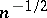for all these spaces, without an additional constant. Hence the problem is tractable in the randomized setting. Many other problems which are intractable in the worst-case setting stay intractable in the randomized or average-case setting.

For results concerning the average-case setting see Bayesian numerical analysis.

How to Cite This Entry:
Curse of dimension. Erich Novak (originator), Encyclopedia of Mathematics. URL: http://encyclopediaofmath.org/index.php?title=Curse_of_dimension&oldid=14053
This text originally appeared in Encyclopedia of Mathematics - ISBN 1402006098